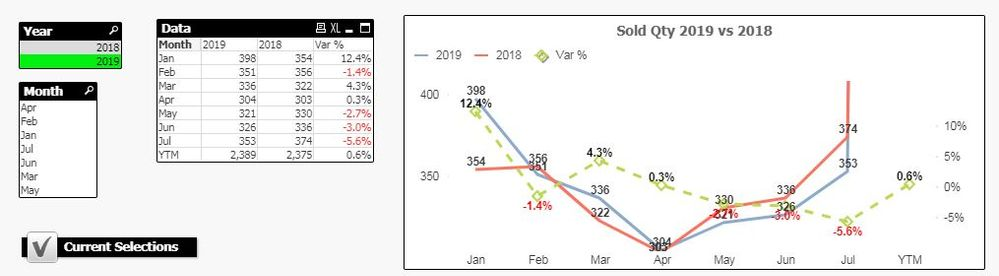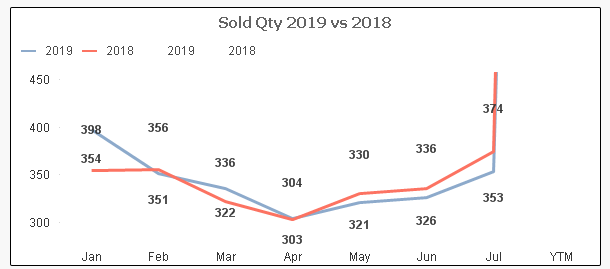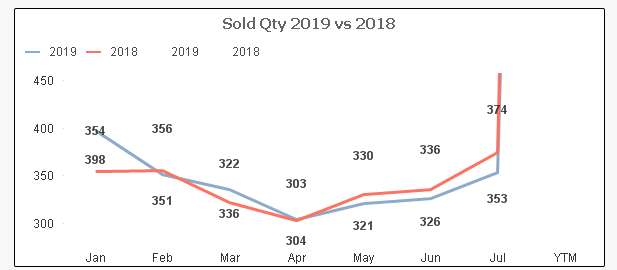# New to QlikView

Discussion board where members can get started with QlikView.

Announcements
Modernize Your QlikView Deployment webinar, Nov. 3rd. REGISTER
cancel
Showing results for
Did you mean:
HighlightedMaster II

## Data points getting overlapped by Line chart in Qlikview

Hi All,

I've a Line graph with three KPI metrics, the issue here is some the values are either getting overlapped by the Line itself or by the data value of another expression value.

Please suggest if any work around to solve this.

Please refer to the mock app with screenshotLabels (1)
• ### line chart

1 Solution

Accepted Solutions
HighlightedMVP

Jan didn't look right where 398 was lower than 354... changed the expressions to this for the display of numbers

``Dual(RangeMin(sum({\$<Year={"\$(=max(Year))"}>}SoldQty), sum({\$<Year={"\$(=max(Year)-1)"}>}SoldQty)), sum({\$<Year={"\$(=max(Year))"}>}SoldQty) * 0.90)``

and

``Dual(RangeMax(sum({\$<Year={"\$(=max(Year))"}>}SoldQty), sum({\$<Year={"\$(=max(Year)-1)"}>}SoldQty)), sum({\$<Year={"\$(=max(Year)-1)"}>}SoldQty) * 1.1)``2 Replies
HighlightedMVP

I simplified your expression from 3 expressions to 2 expressions. Also, this is not perfect, but my solution is based on these two threads

Stopping values on data points overlapping

Values Overlapping on ChartSo, basically I created two new expressions for displaying the value

``Dual(sum({\$<Year={"\$(=max(Year))"}>}SoldQty), sum({\$<Year={"\$(=max(Year))"}>}SoldQty) * 0.90)``
``Dual(sum({\$<Year={"\$(=max(Year)-1)"}>}SoldQty), sum({\$<Year={"\$(=max(Year)-1)"}>}SoldQty) * 1.1)``

and both these lines are set with a background color of ARGB(0, 0, 0, 0). Also, removed 'Value on Data Points' for your actual expressions.

HighlightedMVP

Jan didn't look right where 398 was lower than 354... changed the expressions to this for the display of numbers

``Dual(RangeMin(sum({\$<Year={"\$(=max(Year))"}>}SoldQty), sum({\$<Year={"\$(=max(Year)-1)"}>}SoldQty)), sum({\$<Year={"\$(=max(Year))"}>}SoldQty) * 0.90)``

and

``Dual(RangeMax(sum({\$<Year={"\$(=max(Year))"}>}SoldQty), sum({\$<Year={"\$(=max(Year)-1)"}>}SoldQty)), sum({\$<Year={"\$(=max(Year)-1)"}>}SoldQty) * 1.1)``